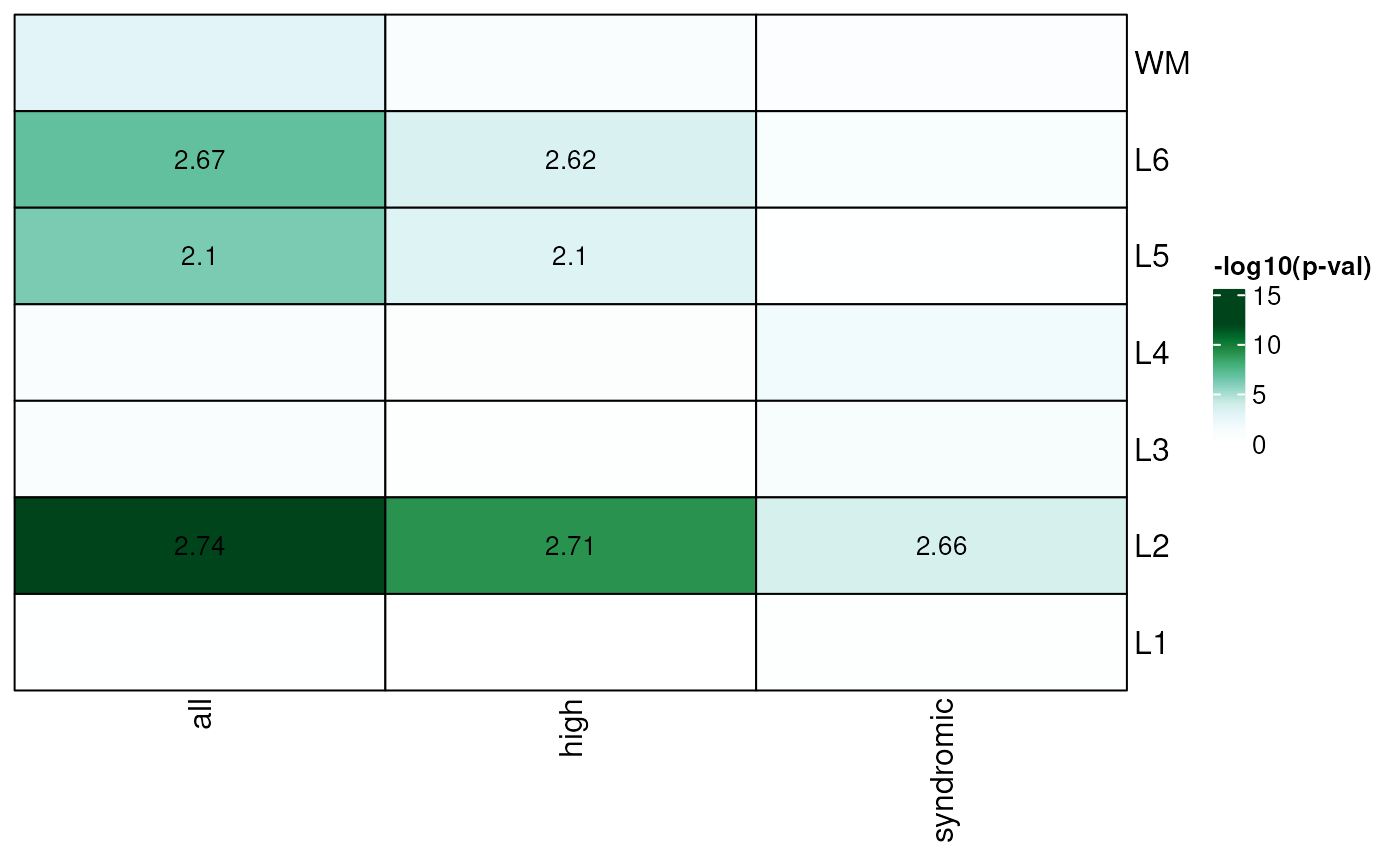This function takes the output of gene_set_enrichment() and creates a heatmap visualization of the results.

gene_set_enrichment_plot(
enrichment,
xlabs = unique(enrichment$ID), PThresh = 12, ORcut = 3, enrichOnly = FALSE, layerHeights = c(0, seq_len(length(unique(enrichment$test)))) * 15,
mypal = c("white", (grDevices::colorRampPalette(RColorBrewer::brewer.pal(9,
"YlOrRd")))(50)),
cex = 1.2
)

## Arguments

enrichment

The output of gene_set_enrichment().

xlabs

A vector of names in the same order and length as unique(enrichment$ID). Gets passed to layer_matrix_plot(). PThresh A numeric(1) specifying the P-value threshold for the maximum value in the -log10(p) scale. ORcut A numeric(1) specifying the P-value threshold for the minimum value in the -log10(p) scale for printing the odds ratio values in the cells of the resulting plot. enrichOnly A logical(1) indicating whether to show only odds ratio values greater than 1. layerHeights A numeric() vector of length equal to length(unique(enrichment$test)) + 1 that starts at 0 specifying where to plot the y-axis breaks which can be used for re-creating the length of each brain layer. Gets passed to layer_matrix_plot().

mypal

A vector with the color palette to use. Gets passed to layer_matrix_plot().

cex

Passed to layer_matrix_plot().

## Value

A plot visualizing the gene set enrichment odds ratio and p-value results.

## Details

Check https://github.com/LieberInstitute/HumanPilot/blob/master/Analysis/Layer_Guesses/check_clinical_gene_sets.R to see a full script from where this family of functions is derived from.

layer_matrix_plot

Other Gene set enrichment functions: gene_set_enrichment()

## Examples


## Read in the SFARI gene sets included in the package
system.file(
"extdata",
"SFARI-Gene_genes_01-03-2020release_02-04-2020export.csv",
package = "spatialLIBD"
),
as.is = TRUE
)

## Format them appropriately
asd_sfari_geneList <- list(
Gene_SFARI_all = asd_sfari$ensembl.id, Gene_SFARI_high = asd_sfari$ensembl.id[asd_sfari$gene.score < 3], Gene_SFARI_syndromic = asd_sfari$ensembl.id[asd_sfari$syndromic == 1] ) ## Obtain the necessary data if (!exists("modeling_results")) { modeling_results <- fetch_data(type = "modeling_results") } #> 2023-09-05 20:31:16.634305 loading file /github/home/.cache/R/BiocFileCache/11447289c394_Human_DLPFC_Visium_modeling_results.Rdata%3Fdl%3D1 ## Compute the gene set enrichment results asd_sfari_enrichment <- gene_set_enrichment( gene_list = asd_sfari_geneList, modeling_results = modeling_results, model_type = "enrichment" ) ## Visualize the gene set enrichment results ## with a custom color palette gene_set_enrichment_plot( asd_sfari_enrichment, xlabs = gsub(".*_", "", unique(asd_sfari_enrichment$ID)),
mypal = c(
"white",
grDevices::colorRampPalette(
RColorBrewer::brewer.pal(9, "BuGn")
)(50)
)
)## Specify the layer heights so it resembles more the length of each
## layer in the brain
gene_set_enrichment_plot(
asd_sfari_enrichment,
xlabs = gsub(".*_", "", unique(asd_sfari_enrichment\$ID)),
layerHeights = c(0, 40, 55, 75, 85, 110, 120, 135),
)